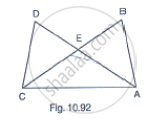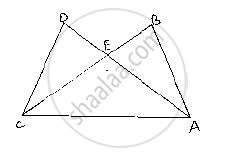Share
Notifications

View all notifications
Books Shortlist
Your shortlist is empty

# In Fig. 10.92, It is Given that Ab = Cd and Ad = Bc. Prove that δAdc ≅ δCba. - CBSE Class 9 - Mathematics

Login
Create free account

Forgot password?
ConceptCriteria for Congruence of Triangles

#### Question

In Fig. 10.92, it is given that AB = CD and AD = BC. Prove that ΔADC ≅ ΔCBA.#### Solution

Given that in the figure AB =CD  and . AD=BC
We have to prove

ΔADC≅ΔCBA

Now,
Consider ΔADC and ΔCBA

We have

AB = CD                 [Given]

BC = AD                 [Given]

And AC=AC             [Common side]

So, by SSS congruence criterion, we have

ΔADC≅ΔCBA

∴ Hence provedIs there an error in this question or solution?

#### APPEARS IN

Solution In Fig. 10.92, It is Given that Ab = Cd and Ad = Bc. Prove that δAdc ≅ δCba. Concept: Criteria for Congruence of Triangles.
S